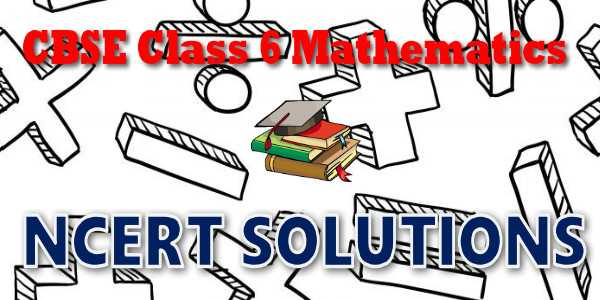# CBSE - Class 06 - Mathematics - Playing with Numbers - NCERT Solutions## CBSE, JEE, NEET, NDA

Question Bank, Mock Tests, Exam Papers

NCERT Solutions, Sample Papers, Notes, Videos

## NCERT Solutions for Mathematics Playing with Numbers## myCBSEguide App

Complete Guide for CBSE Students

NCERT Solutions, NCERT Exemplars, Revison Notes, Free Videos, CBSE Papers, MCQ Tests & more.

# NCERT solutions for class 6  Mathematics Playing with Numbers## NCERT Class 6 Mathematics Chapter-wise Solutions

Maths Part I

• Chapter 1 - Knowing Our Numbers
• Chapter 2 - Whole Numbers
• Chapter 3 - Playing with Numbers
• Chapter 4 - Basic Geometrical Ideas
• Chapter 5 - Understanding Elementary Shapes
• Chapter 6 - Integers
• Chapter 7 - Fractions
• Chapter 8 - Decimals
• Chapter 9 - Data Handling
• Chapter 10 - Mensuration
• Chapter 11 - Algebra
• Chapter 12 - Ratio and Proportion
• Chapter 13 - Symmetry
• Chapter 14 - Practical Geometry

## CBSE class 6th Mathematics have one books. Each book has chapters and topics.

• NCERT Mathematics Book Class 6

Here is the list of topics covered under each chapter of class 6 Mathematics NCERT text book.

• Exercise 1.1
• Exercise 1.2
• Exercise 1.3

• Exercise 2.1
• Exercise 2.2
• Exercise 2.3

• Exercise 3.1
• Exercise 3.2
• Exercise 3.3
• Exercise 3.4
• Exercise 3.5
• Exercise 3.6
• Exercise 3.7

• Exercise 4.1
• Exercise 4.2
• Exercise 4.3
• Exercise 4.4
• Exercise 4.5
• Exercise 4.6

• Exercise 5.1
• Exercise 5.2
• Exercise 5.3
• Exercise 5.4
• Exercise 5.5
• Exercise 5.6
• Exercise 5.7
• Exercise 5.8
• Exercise 5.9

• Exercise 6.1
• Exercise 6.2
• Exercise 6.3

• Exercise 7.1
• Exercise 7.2
• Exercise 7.3
• Exercise 7.4
• Exercise 7.5
• Exercise 7.6

• Exercise 8.1
• Exercise 8.2
• Exercise 8.3
• Exercise 8.4
• Exercise 8.5
• Exercise 8.6

• Exercise 9.1
• Exercise 9.2
• Exercise 9.3
• Exercise 9.4

### 10. Mensuration

• Exercise 10.1
• Exercise 10.2
• Exercise 10.3

### 11. Algebra

• Exercise 11.1
• Exercise 11.2
• Exercise 11.3
• Exercise 11.4
• Exercise 11.5

### 12. Ratio and Proportion

• Exercise 12.1
• Exercise 12.2
• Exercise 12.3

### 13. Symmetry

• Exercise 13.1
• Exercise 13.2
• Exercise 13.3

### 14. Practical Geometry

• Exercise 14.1
• Exercise 14.2
• Exercise 14.3
• Exercise 14.4
• Exercise 14.5
• Exercise 14.6

## NCERT Solutions Chapter 3 Playing with Numbers

Ramesh has 6 marbles with him. He wants to arrange them in rows in such a way that each row has the same number of marbles. He arranges them in the following ways and matches the total number of marbles.
(i) 1 marble in each row
Number of rows = 6
Total number of marbles = 1 × 6 = 6

(ii) 2 marbles in each row
Number of rows = 3

Total number of marbles = 2 × 3 = 6

(iii) 3 marbles in each row

Number of rows = 2

Total number of marbles = 3 × 2 = 6

(iv) He could not think of any arrangement in which each row had 4 marbles or 5 marbles. So,      the only possible arrangement left was with all the 6 marbles in a row.

Number of rows = 1

Total number of marbles = 6 × 1 = 6

From these calculations Ramesh observes that 6 can be written as a product of two numbers    in different ways as

6 = 1 × 6; 6 = 2 × 3; 6 = 3 × 2; 6 = 6 × 1

## NCERT Solutions for Class 6th Mathematics

NCERT Solutions Class 6 Mathematics PDF (Download) Free from myCBSEguide app and myCBSEguide website. Ncert solution class 6 Mathematics includes text book solutions from  NCERT Solutions for CBSE Class 6 Mathematics have total 14 chapters. Class 6 Mathematics ncert Solutions in pdf for free Download are given in this website. Ncert Mathematics class 6 solutions PDF and Mathematics ncert class 6 PDF solutions with latest modifications and as per the latest CBSE syllabus are only available in myCBSEguide.

## NCERT Solutions for Class 5

NCERT Solutions for Class 4

## NCERT Solutions for Class 3## myCBSEguide

Trusted by 1 Crore+ Students

#### CBSE Test Generator

Create papers in minutes

Print with your name & Logo

3 Lakhs+ Questions

Solutions Included

Based on CBSE Blueprint

Best fit for Schools & Tutors

#### Work from Home

• Work from home with us
• Create questions or review them from home

No software required, no contract to sign. Simply apply as teacher, take eligibility test and start working with us. Required desktop or laptop with internet connection